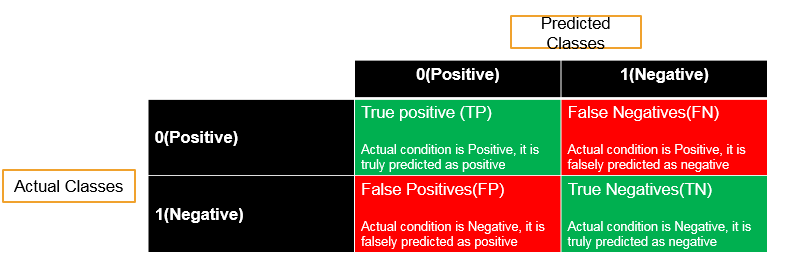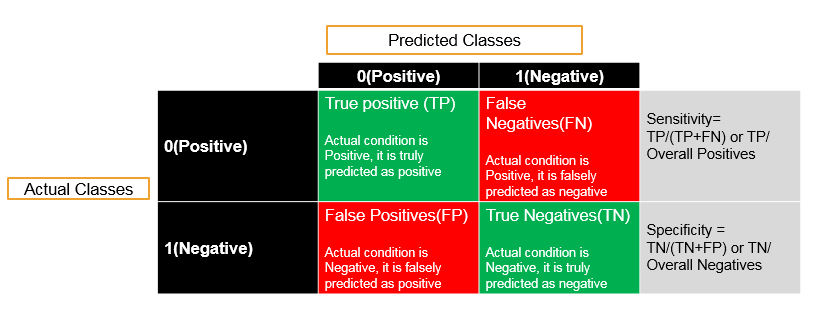• No products in the cart.

# 203.4.1 Model Selection and Cross Validation

##### Starting off with introduction to Model Validation

After learning about the Tree Building Model Selection we will now study about The Model Selection and Cross Validation

In previous series-es we learned the most important and basic algorithms used in analytics industry.

In this series of posts, we will learn how to choose the best model out of many models we have created for specific problem. We will also learn how to improve our per-existing models.

### Model Validation

What is model validation.

• Checking how good is our model.
• It is very important to report the ay of the model along with the final model.
• The model validation in regression is done through R square and Adj R-Square.
• Logistic Regression, Decision tree and other classification techniques have very similar validation measures.
• Till now we have seen confusion matrix and accuracy. There are many more validation and model accuracy metrics for classification models.

### Classification-Validation measures

• Confusion matrix, Specificity, Sensitivity
• ROC, AUC
• KS, Gini
• Concordance and discordance
• Chi-Square, Hosmer and Lemeshow Goodness-of-Fit Test
• Lift curve

All of them are measuring the model accuracy only. Some metrics work really well for certain class of problems. Confusion matrix, ROC and AUC will be sufficient for most of the business problems

### Sensitivity and Specificity

Sensitivity and Specificity are derived from confusion matrix• Accuracy=(TP+TN)/(TP+FP+FN+TN)
• Misclassification Rate=(FP+FN)/(TP+FP+FN+TN)
• Sensitivity : Percentage of positives that are successfully classified as positive
• Specificity : Percentage of negatives that are successfully classified as negativesThe next post is calculating Sensitivity and Specificity in R.

21st June 2017
0 responses on "203.4.1 Model Selection and Cross Validation"
© 2020. All Rights Reserved.